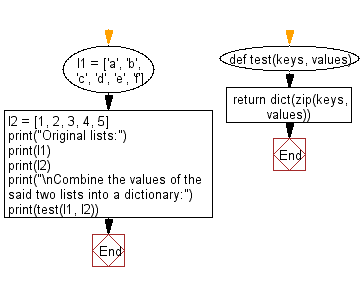﻿ Python: Combine two lists into a dictionary, where the elements of the first one serve as the keys and the elements of the second one serve as the values - w3resource# Python: Combine two lists into a dictionary, where the elements of the first one serve as the keys and the elements of the second one serve as the values

## Python dictionary: Exercise-76 with Solution

Write a Python program to combine two lists into a dictionary, where the elements of the first one serve as the keys and the elements of the second one serve as the values. The values of the first list need to be unique and hashable.

• Use zip() in combination with dict() to combine the values of the two lists into a dictionary.

Sample Solution:

Python Code:

``````def test(keys, values):
return dict(zip(keys, values))

l1 = ['a', 'b', 'c', 'd', 'e', 'f']
l2 = [1, 2, 3, 4, 5]
print("Original lists:")
print(l1)
print(l2)
print("\nCombine the values of the said two lists into a dictionary:")
print(test(l1, l2))
```
```

Sample Output:

```Original lists:
['a', 'b', 'c', 'd', 'e', 'f']
[1, 2, 3, 4, 5]

Combine the values of the said two lists into a dictionary:
{'a': 1, 'b': 2, 'c': 3, 'd': 4, 'e': 5}
```

Flowchart:## Visualize Python code execution:

The following tool visualize what the computer is doing step-by-step as it executes the said program:

Python Code Editor:

Have another way to solve this solution? Contribute your code (and comments) through Disqus.

What is the difficulty level of this exercise?

Test your Programming skills with w3resource's quiz.

﻿

## Python: Tips of the Day

Decapitalizes the first letter of a string:

Example:

```def tips_decapitalize(s, upper_rest=False):
return s[:1].lower() + (s[1:].upper() if upper_rest else s[1:])
print(tips_decapitalize('PythonTips'))
print(tips_decapitalize('PythonTips', True))
```

Output:

```pythonTips
pYTHONTIPS
```